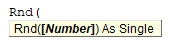# VBA Rnd Function

## Rnd Function

Used to change the seed value used by the random number generator for the Rnd function.

## Simple Rnd Examples

``````Sub Rnd_Example()
Dim randomValue
Randomize

randomValue = Rnd
End Sub
``````

## Rnd Syntax

In the VBA Editor, you can type  “Rnd(” to see the syntax for the Rnd Function:The Rnd function contains an argument:

Number: [Optional] Any valid numeric expression.

## Examples of Excel VBA Rnd Function

To generate random integer value between 1 and 10, you can use the following code.

``````Sub Rnd_Example1()
Dim rndValue As Single
Randomize

For i = 1 To 20
rndValue = Int((Rnd * 10) + 1)
Next i
End Sub``````

This code will generate 20 random integer value between 1 and 10.

To generate random value between an lower bound and an upper bound, you can use the following function.

``````Function RndSpecial(upperbound As Single, lowerbound As Single) As Single
RndSpecial = (upperbound - lowerbound) * Rnd
End Function``````
``````Sub Rnd_Example2()
Dim randomValue As Single
randomValue = RndSpecial(5, 30)
MsgBox randomValue
End Sub``````

This will return a random value between 5 and 30.

Stop searching for VBA code online. Learn more about AutoMacro - A VBA Code Builder that allows beginners to code procedures from scratch with minimal coding knowledge and with many time-saving features for all users!